# Seeing All the Angles: Learning Multiview Manipulation Policies for Contact-Rich Tasks from Demonstrations

### Trevor Ablett, Daniel (Yifan) Zhai, Jonathan Kelly

#### IEEE/RSJ International Conference on Intelligent Robots and Systems (IROS 2021)

Four runs (in real time) of a single multiview policy. The corresponding sensor views are shown at the bottom right.

End-to-end learning has emerged as a popular alternative to the traditional sense-plan-act approach to robotic manipulation. This work was motivated by a relatively simple question: what happens when you try to apply an end-to-end visuomotor policy to a mobile manipulator, in which there is no guarantee that the base will always approach a task from the same relative pose?

We attempted to thoroughly answer this question by conducting experiments with a simulated and a real mobile manipulator. We generated fixed-view and multiview versions of a set of seven challenging and contact-rich tasks and collected human-expert data in each scenario. We then trained a neural network on each dataset, and tested the performance of fixed-view and multiview policies on fixed-view and multiview tasks.

We found that multiview policies, with an equivalent amount of data, not only significantly outperformed fixed-view policies in mulitview tasks, but performed nearly equivalently in fixed-view tasks. This seems to indicate that, given the ability to do so, it may always be worth training multiview policies. We also found that the features learned by our multiview policies tended to encode a higher degree of spatial consistency than those learned by fixed-view policies.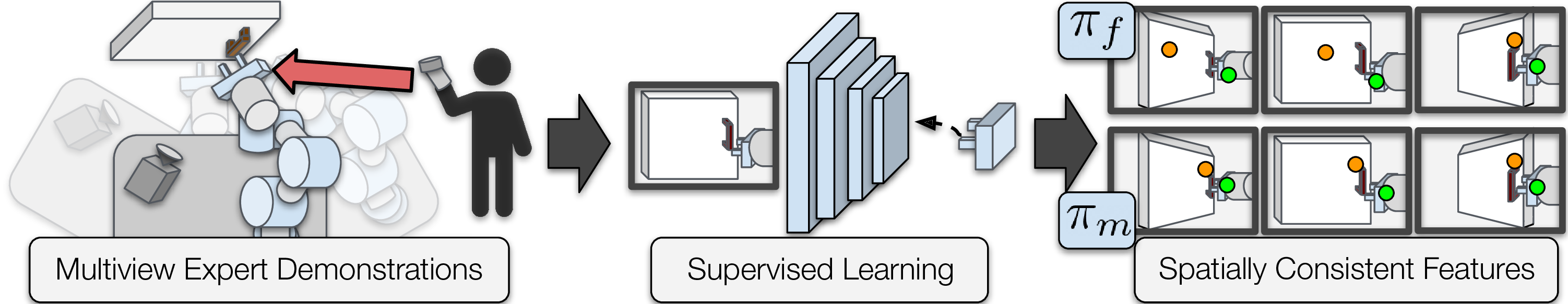The main components of our system for learning multiview policies.

As stated, we completed experiments in seven challenging manipulation tasks.

Autonomous policy execution on the seven tasks used for experiments. Notice that between runs, the base pose changes. These videos are shown at 3x real time.

### Data Collection

We collected data using a human expert for most of our environments using an HTC Vive hand tracker. For our simulated lifting environment, in the interest of creating repeatable experiments, we generated a policy using reinforcement learning. We created a simple method for autonomoulsy choosing randomized base poses between episodes, using:

• approximate calibration between the arm and the base,
• a known workspace center point, and
• approximate localization of the base, which we obtain using wheel odometry alone.

Notably, this information is required only for data collection as a convenience.

A human collecting demonstrations. Notice how the base autonomously resets to new poses between episodes.

### Results

As a reminder, we collected two different datasets for each task: a multiview dataset, where data is collected from the multiview task $$\mathcal{T}_m$$, and a fixed-view dataset, where data is collected from the fixed-view task $$\mathcal{T}_f$$.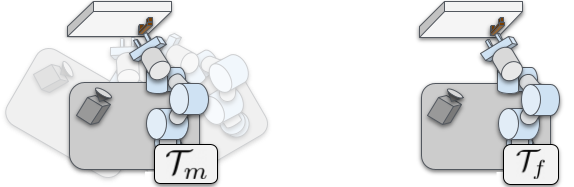In this section, we refer to a multiview policy $$\pi_m$$ and a fixed-view policy $$\pi_f$$ as being trained on $$\mathcal{T}_m$$ and $$\mathcal{T}_f$$ respectively. Because $$\mathcal{T}_m$$ and $$\mathcal{T}_f$$ share an observation and action space, we can test $$\pi_m$$ and $$\pi_f$$ on both $$\mathcal{T}_m$$ and $$\mathcal{T}_f$$!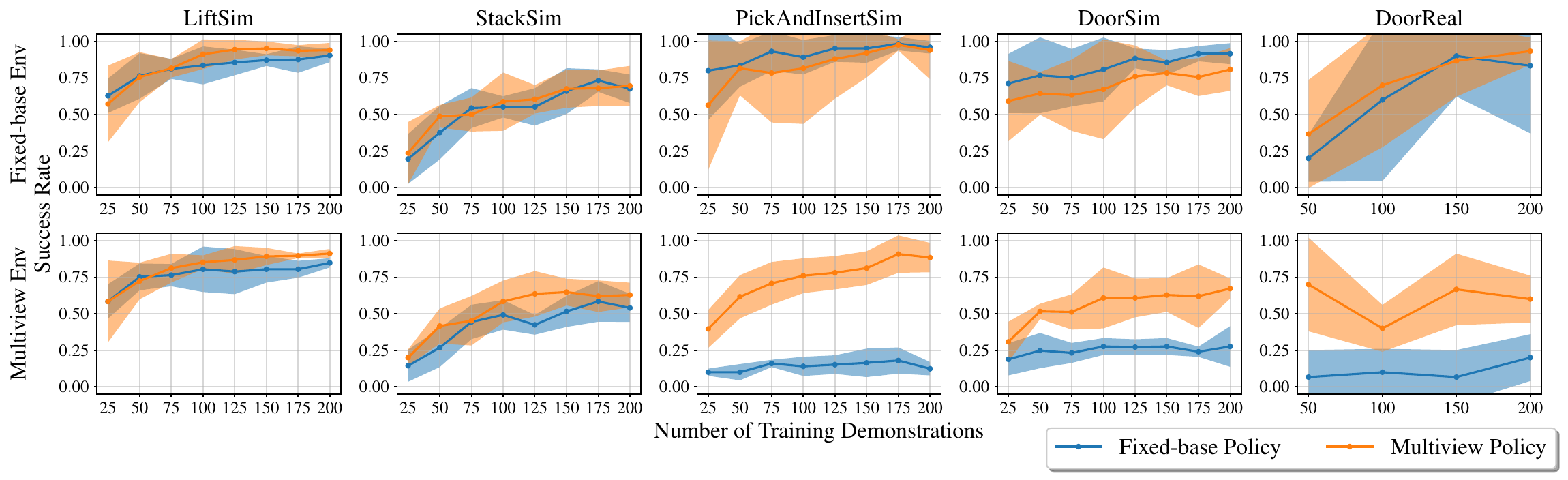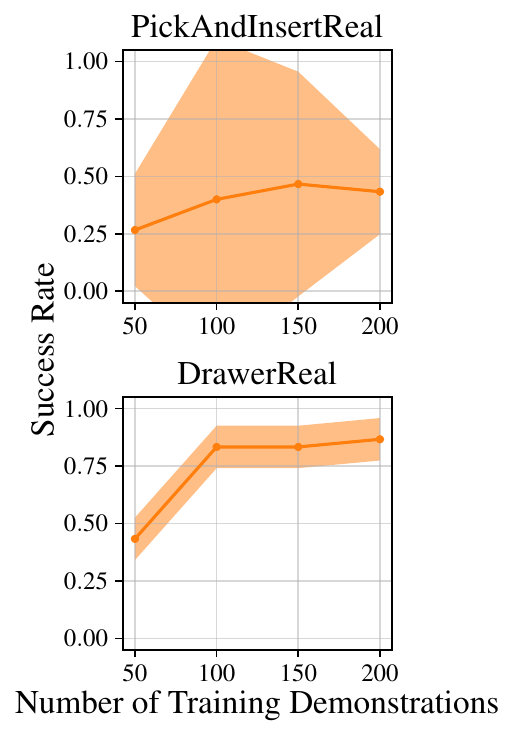The results of $$\pi_m$$ and $$\pi_f$$ in $$\mathcal{T}_m$$ and $$\mathcal{T}_f$$ are shown above, where it is clear that $$\pi_m$$ outperforms $$\pi_f$$ in $$\mathcal{T}_m$$, and, perhaps more surprisingly, $$\pi_m$$ performs comparably to $$\pi_f$$ in $$\mathcal{T}_f$$, despite not having any data at the exact pose used for $$\mathcal{T}_f$$. For PickAndInsertReal and DrawerReal, due to the high potential for environmental damage when running $$\pi_f$$ in $$\mathcal{T}_m$$, we only ran $$\pi_m$$, but we would expect the pattern to be similar to PickAndInsertSim, DoorSim, and DoorReal.

We also showed that $$\pi_m$$ generalizes to out-of-distribution (OOD) data, while, for tasks where mutual information between poses is not generally high (see paper for more details), $$\pi_f$$ performance drops dramatically as soon as the base is moved from $$b_\phi = 0$$, the position at which it was trained.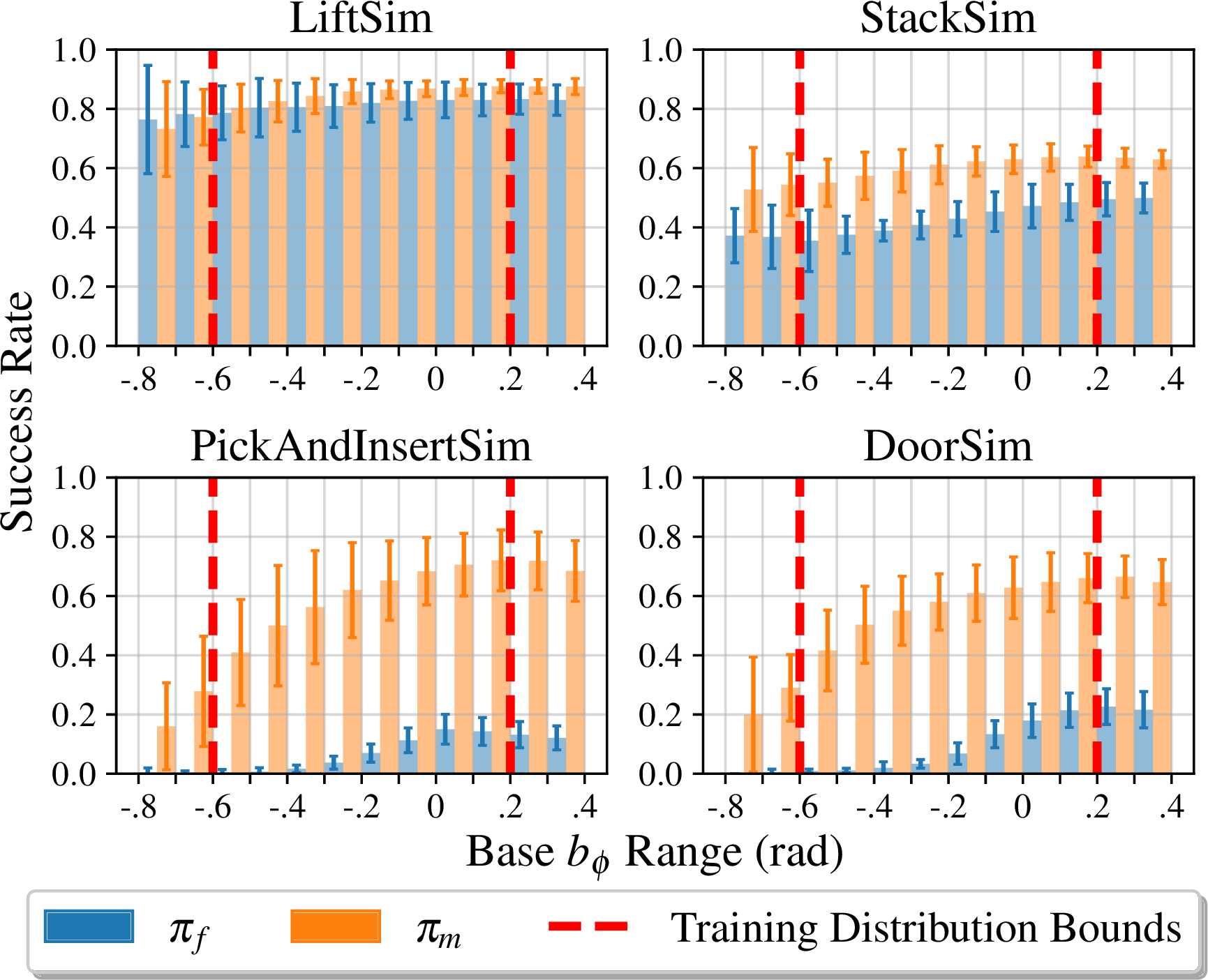### Feature Analysis

Since our network structure includes a spatial-soft argmax layer1, which roughly corresponds to a spatial attention mechnanism, we can analyze the features that it learns.

Two fixed-base policy features.

Each of the features above are the two highest activating features learned by $$\pi_f$$ running in $$\mathcal{T}_m$$. Clearly, they display quite a bit of temporal and spatial spread. The following image shows the highest six activating features learned by $$\pi_f$$:

Six fixed-base policy features.

In contrast, here are the two highest activating features learned by $$\pi_m$$:

Two multiview policy features.

Clearly, the features display more consistency, indicating that the policy, without any loss enforcing it to do so, has learned a degree of view invariance. Once again, here are the six highest activating features:

Six multiview policy features.

For more details, be sure to check out our paper published at IROS 2021!

Coming soon!

## Citation

@inproceedings{ablett_multiview_2021,
AUTHOR    = {Trevor Ablett and Yifan Zhai and Jonathan Kelly},
TITLE     = {Seeing All the Angles: Learning Multiview Manipulation Policies for Contact-Rich Tasks from Demonstrations},
BOOKTITLE = {Proceedings of the {IEEE/RSJ} International Conference on Intelligent Robots and Systems {(IROS'21)}},
YEAR      = {2021},